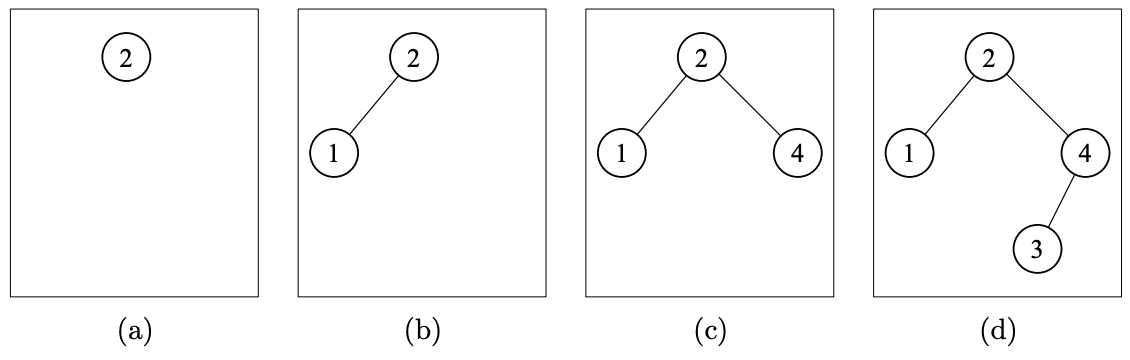시간 제한 메모리 제한 제출 정답 맞은 사람 정답 비율
2 초 512 MB 0 0 0 0.000%

## 문제

A Binary Search Tree (BST) is a binary tree where every node has a value and the tree is arranged so that, for any node all the values in its left subtree are less than the node’s value, and all the values in its right subtree are greater than the node’s value.

To build a BST from a sequence of distinct integers the following procedure is used. The first integer becomes the root of the tree. Then the second integer in the sequence is considered. If it is less than the value of the root node then it becomes the left child. Otherwise, it becomes the right child. Subsequent items in the sequence move down either left or right in the same fashion depending on the comparison against earlier nodes, starting at the root and progressing down the tree. The new node is inserted (as a leaf) into the partially constructed tree when it reaches a missing subtree in the particular direction of travel.

For example, a BST generated by the sequence [2, 1, 4, 3] is built up as shown below as the numbers are inserted.Figure 3: Generation of BST

The tree (d) of Figure 3 can also be generated by two other sequences: [2, 4, 3, 1] and [2, 4, 1, 3].

Such sequences can be compared according to a lexicographic order, where the comparison proceeds left-to-right and items at corresponding positions are compared using their numerical values. The result for [2, 1, 4, 3], [2, 4, 3, 1] and [2, 4, 1, 3] is as follows, using “<” to denote this lexicographic order between sequences: [2, 1, 4, 3] < [2, 4, 1, 3] < [2, 4, 1, 3].

For the tree (d) in Figure 3 the lexicographically least sequence is [2, 1, 4, 3].

Write a program that will read a representation of a tree and will generate the lexicographically least sequence of distinct positive integers that will generate that tree. Note that for a tree with n nodes this sequence will be a permutation of the numbers from 1 to n.

For the input to our problem we represent (recursively) the structure of a tree as follows:

1. A single node (a leaf) is a tree:
• ()
2. If TL and TR are trees then the following are also trees:
• (TL,)
• (,TR)
• (TL,TR)

## 입력

Input will consist of a sequence of lines. Each line will consist of up to 250 characters and will contain the specification of a single tree according to the above definition (with no intervening spaces). The sequence will be terminated by a tree consisting of a single node (). This line should not be processed.

## 출력

Output will be a sequence of lines, one for each line in the input. Each line will consist of the required permutation of the integers from 1 to n (where n is the number of nodes in the tree) separated by single spaces.

## 예제 입력 1

((),((),))
(((),),())
(((),()),((),()))
(,())
((),)
()


## 예제 출력 1

2 1 4 3
3 2 1 4
4 2 1 3 6 5 7
1 2
2 1